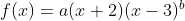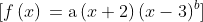Scan QR code or get instant email to install app

Question:

# 1212In the function, a and b are both integer constants and b is positive. If the end behavior of the graph of y = f(x) is positive for both very large negative values of x and very large positive values of x, what is true about a and b?

A a is positive, and b is odd.
explanation

1212A polynomial function of even degree with a positive leading coefficient will have positive end behavior for both very large negative values of x and very large positive values of x. For a polynomial function in the formto be of even degree with a positive leading coefficient, a must be positive and b must be odd.